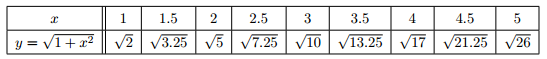# Trapezoidal Rule Formula

In mathematics, and more specifically in numerical analysis, the trapezoidal rule, also known as the trapezoid rule or trapezium rule, is a technique for approximating the definite integral.

The trapezoidal rule works by approximating the region under the graph of the function f(x) as a trapezoid and calculating its area.

The trapezoidal rule is to find the exact value of a definite integral using a numerical method. This rule is mainly based on the Newton-Cotes formula which states that one can find the exact value of the integral as an nth order polynomial.

When n = 1 according to Trapezoidal rule, the area under the linear polynomial is stated as,

$\large \int_{a}^{b}f\left ( x \right )dx=\left ( b-a \right )\left [ \frac{f\left ( a \right )+f\left ( b \right )}{2} \right ]$

### Solved Examples of Trapezoidal Rule

Example 1: Use the trapezoidal rule with n = 8 to estimate:

$\int_{1}^{5}\sqrt{1+x^{2}}dx$

Solution:

Given,

For n=8, we have, $\Delta x=\frac{5-1}{8}=0.5$ we compute the values of $y_{0}$, $y_{1}$, $y_{2}$, . . . , $y_{8}$.Therefore,

$\int_{1}^{5}\sqrt{1+x_{2}}dx\approx \frac{0.5}{2}\left (\sqrt{2}+\sqrt{3.25}+\sqrt{5}+\sqrt{7.25}+\sqrt{10}+\sqrt{13.25}+\sqrt{17}+\sqrt{21.25}+\sqrt{26}\right)$

$\approx 12.76$

#### 1 Comment

1. Pancrasio lukelo

I like it